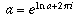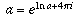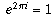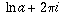Navigation Panel:(These buttons explained below)Question Corner and Discussion Area

# Raising a Number to a Complex Power

Asked by Wei-Nung Teng, student, Stella Matutina Girl's High School on June 17, 1997:
How do you define a^(b+ci)? a, b, c are real numbers.

example: 5^(3+2i)=?

Thanks

You can find a detailed answer to this question by reading the answers to some of the other questions on this site, but here is a summary of the answer all together in one place.

The ordinary definition of exponentiation of real numbers (a^x) only makes sense when x is rational. To extend the definition to irrational and then to complex values of x, you need to rewrite the definition in a way that makes sense even when r is complex.

One way to do this is to use the fact that e^x can be expressed as the infinite sum(where n! means n factorial, the product of the numbers 1,2,. . . ,n).

It makes perfectly good sense to add and multiply complex numbers, and the theory about infinite sums can also be extended to complex numbers, so this formula can be used as a definition of what e^x means when x is complex.

If x is a "purely imaginary" number, that is, if x=ci where c is real, the sum is very easy to evaluate, using the fact that i^2=-1, i^3=-i, i^4=1, i^5=i, etc. When you do this and split the sum into its real and imaginary parts, you find that the real part is the same as the infinite sum expression for cos c, and the imaginary part is the same as the infinite sum expression for sin c. This gives rise to de Moivre's formula:

e^(ic) = (cos c) + i(sin c)
This is explained in more detail in the answer to another question. Also given in that answer is an alternative explanation which doesn't rely on the fact that functions like e^x, cos x, and sin x have representations as infinite sums. That's a fact you probably haven't seen yet, and it's hard to explain without some calculus background.

Now we know what e raised to an imaginary power is. One can also show that the definition of e^x for complex numbers x still satisfies the usual properties of exponents, so we can find e to the power of any complex number b + ic as follows:

e^(b+ic) = (e^b)(e^(ic)) = (e^b)((cos c) + i(sin c))
Finally, for a real number a, you can define a^(b+ic) by writing a = e^(ln a):This answers the question you asked. Now, if a is a complex number instead of a real number, things are more complicated. There is no single value to "ln a": there are lots of different complex numbers z for which e^z = a, and for any such complex number z, you could define a^(b+ic) to be e^(z(b+ic)) and use the above technique to calculate it. This is illustrated in the answer to another question, where it is shown that the expression i^i has an infinite set of possible values.

In fact, the same thing is true even when a is a real number. Technically speaking, the expression a^(b+ic) has infinitely many possible values (except when b and c are both rational numbers), because instead of doing the calculation writing a = e^(ln a), you could also do it by writing, or by writing, or, and so on. Each of these equalities is true (you can check them using de Moivre's formula to show that, so adding multiples ofto the exponent is the same as multiplying by 1, which doesn't affect the truth of the equality). Using these different equalities to do the calculation gives rise to infinitely many different possible values for a^(b+ci).

When a is real it is more "natural" to use the ordinary real-valued logarithm ln a rather than than something like. So it is reasonable to think of a^(b+ic) as having only one value (in much the same way as we think of 4^(1/2) as equalling 2 even though both 2 and -2 are square roots of 4). Technically, this value is called the principal value. This is what the formula up above gives you.

However, when a is not real there is no one natural choice of logarithm to prefer over any other, so in those cases we have to say that an expression like a^(b+ic) has many different values.

[ Submit Your Own Question ] [ Create a Discussion Topic ]

This part of the site maintained by (No Current Maintainers)
Last updated: April 19, 1999
Original Web Site Creator / Mathematical Content Developer: Philip Spencer
Current Network Coordinator and Contact Person: Joel Chan - mathnet@math.toronto.edu

Navigation Panel: